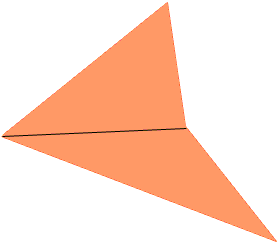Quandaries and Queries I want to know how to find the area of a quadrilateral. Hi Jonathan, The way to find the area of a quadrilateral is to divide it into two triangles and add the areas of the triangles.The area of a triangle you can find either by the familiar "half the base times the height" expression or by using Heron's Formula. Penny Go to Math Central